# Morphology¶

Morphology modifies an image by evaluating the pixel values surrounding each pixel. The basic Wand method signature is:

```img.morphology(method, kernel, iterations)
```

Where `method` is the operation to apply, and is defined by `MORPHOLOGY_METHODS`. The `kernel` can include predefined built-in shapes, or user-defined shapes.

## Shapes¶

Shapes, also known as “kernels”, are small matrices that control morphology method operations. The kernels define the size, and targeted pixels to modify.

To demonstrate a kernel’s shape; let’s generate a simple black canvas around a white pixel.

```from wand.image import Image

with Image(width=1, height=1, pseudo='xc:white') as img:
img.border('black', 6, 6, compose='copy')
img.save(filename='morph-dot.png')
```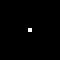### Built-In Kernels¶

ImageMagick contains about three dozen pre-built kernels that cover most common morphology uses, as well as a few specific ones leveraged for internal operations. To use built-in kernels, the following string format is required.

```label[:arg1,arg2,arg3,..]
```

Where label is a string defined in `KERNEL_INFO_TYPES`. Each label can have additional optional arguments, which are defined by a comma separated list of doubles. A colon `':'` symbol should separate the label & argument list. For example:

```disk:2.5,3,5
```

Below is a small list of examples for the most common kernel shapes.

#### Cross¶

```with Image(filename='morph-dot.png') as img:
img.morphology(method='dilate', kernel='cross:3')
img.sample(width=60, height=60)
img.save(filename='morph-kernel-cross3.png')
```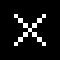#### Diamond¶

```with Image(filename='morph-dot.png') as img:
img.morphology(method='dilate', kernel='diamond:3')
img.sample(width=60, height=60)
img.save(filename='morph-kernel-diamond3.png')
```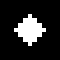#### Disk¶

```with Image(filename='morph-dot.png') as img:
img.morphology(method='dilate', kernel='disk:5')
img.sample(width=60, height=60)
img.save(filename='morph-kernel-disk5.png')
```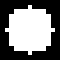#### Octagon¶

```with Image(filename='morph-dot.png') as img:
img.morphology(method='dilate', kernel='octagon:5')
img.sample(width=60, height=60)
img.save(filename='morph-kernel-octagon5.png')
```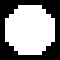#### Plus¶

```with Image(filename='morph-dot.png') as img:
img.morphology(method='dilate', kernel='plus:3')
img.sample(width=60, height=60)
img.save(filename='morph-kernel-plus3.png')
```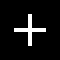#### Ring¶

```with Image(filename='morph-dot.png') as img:
img.morphology(method='dilate', kernel='ring:5,4')
img.sample(width=60, height=60)
img.save(filename='morph-kernel-ring5.png')
```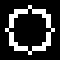#### Square¶

```with Image(filename='morph-dot.png') as img:
img.morphology(method='dilate', kernel='square:3')
img.sample(width=60, height=60)
img.save(filename='morph-kernel-square3.png')
```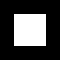### Custom Kernels¶

Users can define their own kernel shape by building a string that follows the format:

```geometry:pix1,pix2,pix3,...
```

Where geometry is defined as WIDTHxHEIGHT of the kernel, followed by a colon, and then a comma separated list of float values. For example:

```custom_kernel = """
5x5:
-,-,1,-,-
-,1,1,1,-
-,-,-,-,-
-,1,-,1,-
1,1,1,1,1
"""

with Image(filename='morph-dot.png') as img:
img.morphology(method='dilate', kernel=custom_kernel)
img.sample(width=60, height=60)
img.save(filename='morph-kernel-custom.png')
```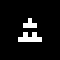By default, the kernel’s “origin” is calculated to be at the center of the kernel. Users can set the kernel origin by defining ±X±Y as part of the geometry. For example:

```top_left_origin = """
3x3+0+0:
1,1,-
1,0,0
-,0,-
"""

bottom_right_origin = """
3x3+2+2:
1,1,-
1,0,0
-,0,-
"""
```

## Methods¶

Morphology methods are broken into three general groups. Basic methods (such as Erode, Dilate, Open, & Close) are used to increase or reduce foreground shapes. Difference methods (such as Edge In, Edge Out, Top Hat & Bottom Hat) draw pixels around foreground edges. Pattern matching methods (such as Hit and Miss, Thinning & Thicken) add pixels when a kernel is matched.

Morphology is intended for images with a black background, and a white foreground. To demonstrate morphology methods, let’s create a basic binary image. We can quickly generate a PBM image from bytes-string literal.

```pbm = b"""P1
10 10
1 1 1 1 1 1 1 1 1 1
1 1 0 1 1 0 0 0 0 1
1 0 0 0 1 0 0 1 1 1
1 1 0 1 1 0 0 0 0 1
1 1 1 1 1 0 0 1 1 1
1 1 1 1 0 0 0 0 0 1
1 0 0 0 0 0 0 1 1 1
1 0 1 0 1 0 1 1 1 1
1 0 1 0 1 0 1 1 0 1
1 1 1 1 1 1 1 1 1 1
"""

with Image(blob=pbm, format="PBM") as img:
img.sample(100, 100)
img.save(filename="morph-src.png")
```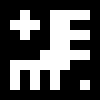The morphology examples below will all use `'morph-src.png'` source image.

### Erode¶

Erode reduces matching white pixels, and expands black spaces.

```with Image(filename='morph-src.png') as img:
img.morphology(method='erode', kernel='octagon')
img.save(filename='morph-erode.png')
```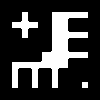### Dilate¶

Dilate increases matching white pixels, and reduces black spaces.

```with Image(filename='morph-src.png') as img:
img.morphology(method='dilate', kernel='octagon')
img.save(filename='morph-dilate.png')
```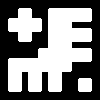### Open¶

Open rounds the white edges, but preserves “holes”, or black corners.

```with Image(filename='morph-src.png') as img:
img.morphology(method='open', kernel='octagon')
img.save(filename='morph-open.png')
```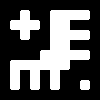Notices the black “inner” corners remain sharp, but the white “outer” corners are rounded.

### Close¶

Close rounds the black edges, and removes any “holes”.

```with Image(filename='morph-src.png') as img:
img.morphology(method='close', kernel='octagon')
img.save(filename='morph-close.png')
```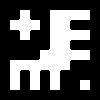Notices the white “outer” corners remain sharp, but the black “inner” corners are rounded.

### Smooth¶

Smooth applies both Open & Close methods. This will remove small objects & holes, and smooth both white & black corners.

```with Image(filename='morph-src.png') as img:
img.morphology(method='smooth', kernel='octagon')
img.save(filename='morph-smooth.png')
```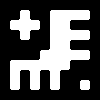### Edge In¶

Edge In method performs a Erode, but only keeps the targeted pixel next to a shape. This means the edge is drawn just inside the white of a object.

```with Image(filename='morph-src.png') as img:
img.morphology(method='edgein', kernel='octagon')
img.save(filename='morph-edgein.png')
```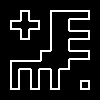### Edge Out¶

Edge Out performs similar to Edge In, but uses the results of Dilate to draw a edge border just outside of an object.

```with Image(filename='morph-src.png') as img:
img.morphology(method='edgeout', kernel='octagon')
img.save(filename='morph-edgeout.png')
```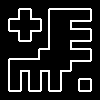### Edge¶

The Edge method performs both Erode & Dilate methods, but only keeps differences between them as the resulting image. The result is border drawn on the edge of the objects within the image.

```with Image(filename='morph-src.png') as img:
img.morphology(method='edge', kernel='octagon')
img.save(filename='morph-edge.png')
```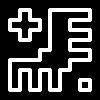### Top Hat¶

The Top Hat method performs the Open morphology method, but only returns the pixels matched by the kernel.

```with Image(filename='morph-src.png') as img:
img.morphology(method='tophat', kernel='octagon')
img.save(filename='morph-tophat.png')
```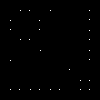### Bottom Hat¶

The Bottom Hat method performs the Close morphology method, but only returns the pixels matched by the kernel.

```with Image(filename='morph-src.png') as img:
img.morphology(method='bottom_hat', kernel='octagon')
img.save(filename='morph-bottom_hat.png')
```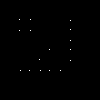### Hit and Miss¶

The hit-and-miss (a.k.a. HMT) method will remove all pixels from the image, unless a kernel pattern is matched; in which case, the pixel under the matched kernel will be set to white.

```with Image(filename='morph-src.png') as img:
corners = """
3x3:
1,1,-
1,0,0
-,0,-
"""
img.morphology(method='hit_and_miss', kernel=corners)
img.save(filename='morph-hit_and_miss.png')
```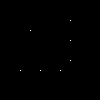### Thinning¶

The thinning method removes a pixel when the kernel matches neighboring pixels. When using custom kernels, you can control which pixel should be targeted by setting the X/Y offset of the kernel’s geometry.

```with Image(filename='morph-src.png') as img:
img.morphology(method='thinning',
kernel='3x1-0-0:1,1,0',
iterations=3)
img.save(filename='morph-thinning.png')
```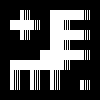There’s also a special `'skeleton'` built-in kernel, paired with -1 iterations to continue to reduce all pixels down to a minimum line.

```with Image(filename='morph-src.png') as img:
img.morphology(method='thinning',
kernel='skeleton',
iterations=-1)
img.save(filename='morph-thinning-skeleton.png')
```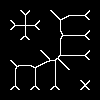### Thicken¶

The thicken method adds a pixel whenever a kernel matches neighboring pixels. You can control the targeted pixel by defining the offset geometry on custom kernels.

```with Image(filename='morph-src.png') as img:
K = """
3x3+0+0:
0,-,-
-,0,-
-,-,1
"""
img.morphology(method='thicken',
kernel=K,
iterations=4)
img.save(filename='morph-thicken.png')
```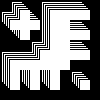### Distance¶

Distance method is a unique, and very special morphology. Given a binary black & white image, each white pixel will be replace with a color value corresponding to the distance to the nearest edge.

```with Image(filename='morph-src.png') as img:
img.morphology(method='distance',
kernel='euclidean',
iterations=4)
img.save(filename='morph-distance-raw.png')
```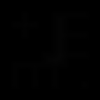The resulting image is not that special. The pixel values are so low that they appear black. However, if we use `auto_level()` method, we should be able to shift the values across the full grayscale.

```with Image(filename='morph-src.png') as img:
img.morphology(method='distance',
kernel='euclidean',
iterations=4)
img.auto_level()
img.save(filename='morph-distance-auto.png')
```Other kernels used for distance morphology are `'chebyshev'`, `'manhattan'`, `'octagonal'`, and `'euclidean'`. The basic kernel string format is:

```distance_kernel[:radius[,scale]]
```

For example:

```manhattan:5,400
```